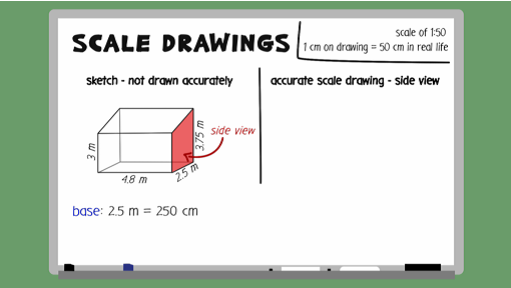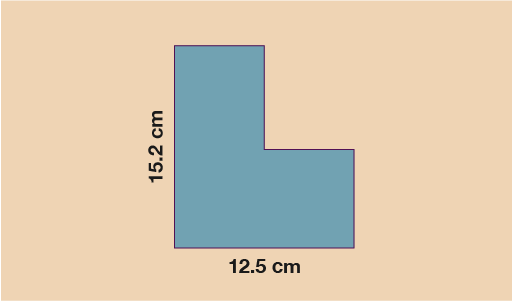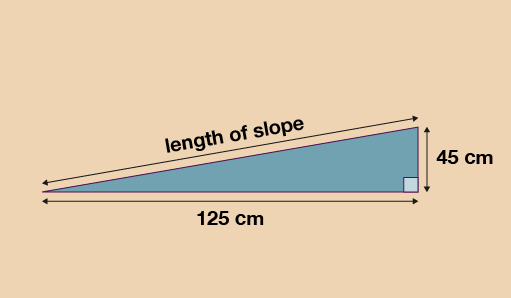Science, Maths & Technology

### Become an OU studentEveryday maths 2

Start this free course now. Just create an account and sign in. Enrol and complete the course for a free statement of participation or digital badge if available.

# 4 Scale drawings and plans

Given that you already have the knowledge you need around measures and ratios, scale drawings are simply just another application of these skills.Interactive feature not available in single page view (see it in standard view).

If you are given the scale drawing and asked to work out the real-life size, rather than dividing by the scale, you multiply by it. Take a look at the example below.

## Case study _unit5.4.1 Example: Calculating scale

A scale drawing has been drawn below of a shed that a garden planner wants to build. The scale used for the drawing is 1:20. The area that the shed will be built on is a rectangle which measures 3.1 m by 2.8 m.

Will the building fit into the space allocated?Figure _unit5.4.1 Figure 37 Calculating scale

### Method

Since 1 cm on the diagram represents 20 cm in real life, you do:

#### Extract _unit5.4.1

Horizontal length: 12.5 × 20 = 250 cm = 2.5 m

Vertical length: 15.2 × 20 = 304 cm = 3.04 m

Since both lengths for the shed are shorter than the lengths given for the area of land, you know the shed will fit.

Now have a go at an example for yourself.

## Activity _unit5.4.1 Activity 8: Finding the length of a slope

For this question you will need a pen or pencil, paper and a ruler.

Jane has a raised vegetable patch. She plans to build a slope leading up to the vegetable patch. Jane will cover the slope with grass turf.

She draws this sketch of the cross section of the slope.Figure _unit5.4.2 Figure 38 Finding the length of a slope

Jane will use a scale diagram to work out the length of the slope. She wants to use a scale of 1:10.

Draw a scale diagram of the slope for Jane. Use it to find the length of the slope.

Base of patch = 125 ÷ 10 = 12.5 cm

Height of patch = 45 ÷ 10 = 4.5 cm

The length of the slope on your drawing should therefore measure around 13.2 cm and when you use the scale of 1:10:

• 13.2 × 10 = 132 cm or 1.32 m in real life

Answers in the range of 1.30 m to 1.36 m are considered accurate and correct.

## Summary

In this section you have:

• applied your ratio skills to the concept of scale plans and drawings

• interpreted scale plans and decided upon a suitable scale for a drawing.